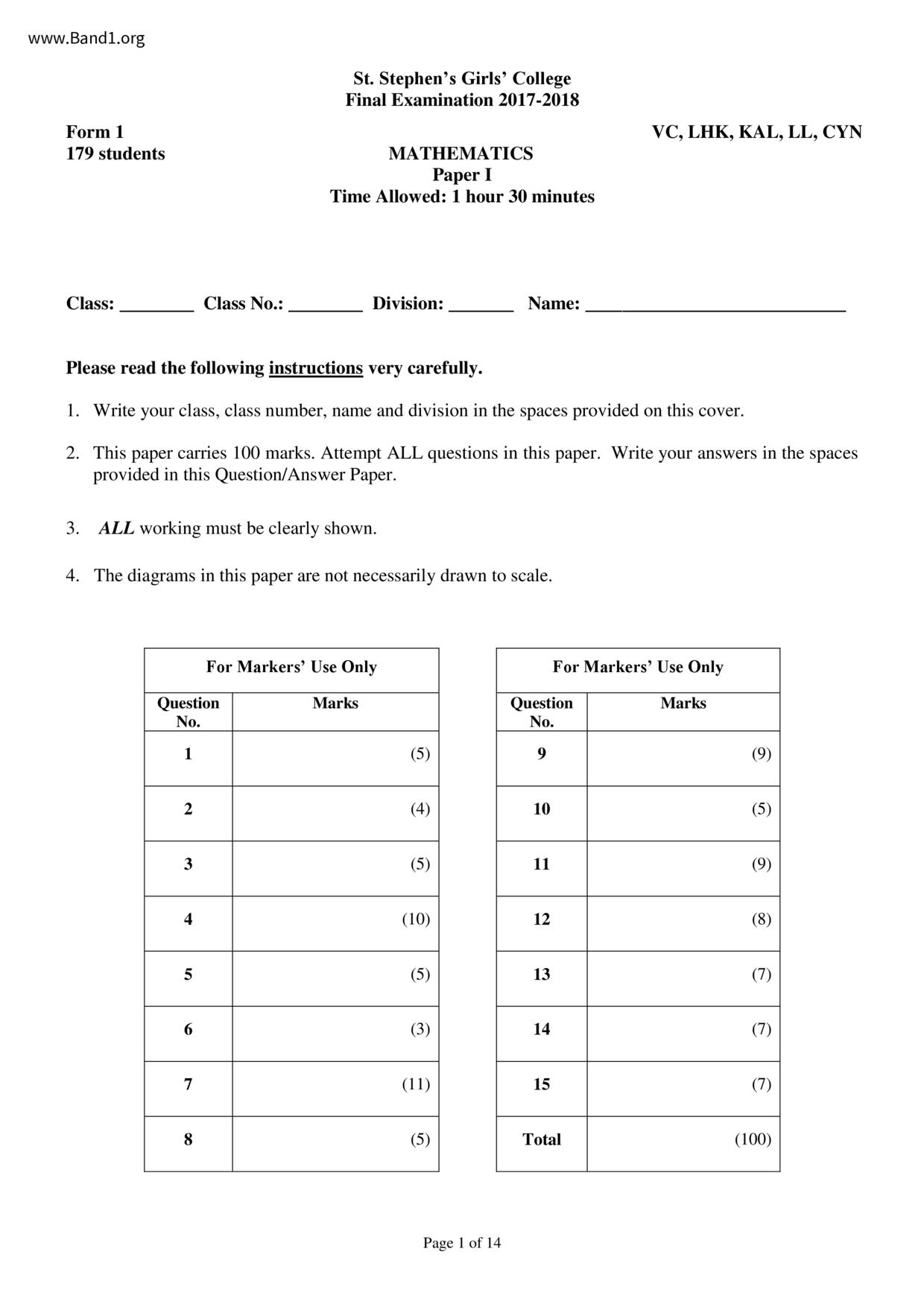# 中一 數學試卷 (F1 Maths Past Paper)

6937

pdf

14

-Ma-P1-17-18-fy

▼ 圖片只作預覽, 如欲下載整份卷, 請按「免費成為會員」 ▼▲ 圖片只作預覽, 如欲下載整份卷, 請按「免費成為會員」 ▲

## 中一數學試卷 PDF 下載

179 students
1. Write your class, class number, name and division in the spaces provided on this cover.
2. This paper carries 100 marks. Attempt ALL questions in this paper. Write your answers in the spaces
ALL working must be clearly shown.
4. The diagrams in this paper are not necessarily drawn to scale.
St. Stephen's Girls' College
Final Examination 2017-2018
MATHEMATICS
Time Allowed: 1 hour 30 minutes
For Markers' Use Only
Page 1 of 14
VC, LHK, KAL, LL, CYN
For Markers' Use Only
F.1 Mathematics Paper I Final Examination 2017-2018
11. The figure shows a rectangular coordinate plane. P(-4, 3), Q(2, 4), R(5, −3) and S(-4, -2) are four
points in the rectangular coordinate plane.
(a) QS intersects the y-axis at N. Write down the coordinates of N.
Find the area of PQRS.
Let M be the point of intersection of the diagonals of PQRS. Mark M in the rectangular
coordinate plane and write down the coordinates of M.
Page 10 of 14
F.1 Mathematics Paper I Final Examination 2017-2018
12. In the figure, ROP and RST are straight lines. It is given that ZQSU = 3ZUST, QS // PU, ZR = 21°
and ZP = 63°.
(a) Find ZUST.
(b) Is QP parallel to SU? Give reasons.
Page 11 of 14
F.1 Mathematics Paper I Final Examination 2017-2018
13. In the figure, XYV and PUYQ are straight lines. It is given that PQ // ST and SU // TV. Find a and b.
Page 12 of 14
F.1 Mathematics Paper I Final Examination 2017-2018
14. In the figure, D is a point on AB. It is given that ZCAD = ZBCD, BC = 8 and BD = 4.
(a) Prove that AABC~ ACBD.
(b) Find the length of AD.
Page 13 of 14
F.1 Mathematics Paper I Final Examination 2017-2018
15. In the figure, RQ // ST. It is given that ZPRT = 160°.
(a) Find ZPRQ.
(b) Is it true that APRQ = ATRS? Give reasons.
* End of Paper *
Page 14 of 14
F.1 Mathematics Paper I Final Examination 2017-2018
1. The height H cm of a plant after n years can be calculated by the formula H =
(a) Find the height of the plant after 6 years.
(b) If H= 90, find n.
2. Mary has distributed at least 130 sweets to n boys and (n-3) girls. Each boy gets 4 sweets and each
girl gets 6 sweets.
(a) Use an inequality to represent the above situation.
(b) Write down two possible values of n.
Page 2 of 14
F.1 Mathematics Paper I Final Examination 2017-2018
3. Solve the equation
Page 3 of 14
F.1 Mathematics Paper I Final Examination 2017-2018
4. The figure shows a pie chart representing the monthly expenditure of Mr. Wong. It is given that he
spends \$7 800 on food and \$9 000 on rent in a month.
Monthly Expenditure of Mr. Wong
Transportation/
Page 4 of 14
(a) Find the total monthly income of Mr. Wong.
(b) Find the values of x and y.
(c) What percentage of Mr. Wong's monthly income is his spending on food?
F.1 Mathematics Paper I Final Examination 2017-2018
5. Listed below are the sizes (in sq. ft.) of 15 different flats in a newly developed residential building:
Use 10 sq. ft. as the stem and 1 sq. ft. as the leaf, construct a stem-and-leaf diagram to present the
above data.
6. The following figure consists of three semi-circles. Find the area of the shaded region and give your
answer correct to 1 decimal place.
Page 5 of 14
F.1 Mathematics Paper I Final Examination 2017-2018
7. Prism A is a metal block in the shape of a rectangular prism. It is melted and recast to form another
prism B. The base of prism B is a right-angled triangle.
(a) (i) Find the volume of prism A.
Find the value of h.
Page 6 of 14
Find the total surface area of prism A.
Find the length of BC.
(iii) Do prism A and prism B have equal total surface area? Explain your answer.
F.1 Mathematics Paper I Final Examination 2017-2018
8. In the figure, AABC is a right-angled triangle.
(a) Draw the image AA₁B₁C₁ after reflecting AABC in the straight line L₁.
(b) Draw the image AA2B₂C2 after rotating AABC clockwise about O through 180°.
(c) According to the sizes of its angles, what kind of triangle is AA1C1C₂?
Page 7 of 14
F.1 Mathematics Paper I Final Examination 2017-2018
9. In the figure, the coordinates of the points A and B are (-5, 3) and (-5, 4) respectively. A is rotated
clockwise about the origin O through 270° to P. B is translated 8 units to the right and then translated 9
units downwards to R.
(a) Find the coordinates of P and R.
(b) If P undergoes a transformation to R, describe the transformation.
(c) Find the area of AOPR.
(d) Q and S are two points in the rectangular coordinate plane such that PQRS has more than one axis
of symmetry. Write down a possible set of coordinates for Q and S.
Page 8 of 14
F.1 Mathematics Paper I Final Examination 2017-2018
10. The figure shows a polar coordinate plane. Denote O as the pole. The coordinates of a point S are
(5, 200⁰).
(a) Plot S in the polar coordinate plane.
(b) Write down the coordinates of R.
(c) According to the sizes of its angles, what kind of triangle is AORS? Explain your answer.
110° 100° 90°
250 260 270 280°
Page 9 of 14
1 2 3 4 5 6